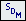# SDM | Calculate New ModesCalculates new modes for a structure using the Structural Dynamics Modification (SDM) method.  The following items are required before using this command,

1. A Shape Table containing UMM mode shapes for the unmodified structure

2. A structure model with FEA Objects attached that model the structural modifications

3. Engineering units in the Structure window that match the units of the UMM mode shapes

The UMM mode shapes must animate correctly on the structure model before using them with this command.

## UMM Mode Shape and Structure Units

• The Structure window Force & Length units (chosen on the Units tab in the File | Structure Options box), must match the Force & Length units of the UMM mode shapes.

• SDM Mass modifications are scaled to make their units compatible with the Force & Length units of the UMM mode shapes.

## FEA Objects

FEA properties that can be used with the SDM to model structural modifications are listed in the Table below.

See the FEA Objects section in this chapter for details of adding FEA Objects to a model.

 FEA Object Object Properties Spring Stiffness Damper Damping Mass Mass Rod cross sectional Area Elasticity, Density Bar cross sectional Area X inertia, Y inertia, XY inertia Elasticity, Density Triangular Plate Thickness, Elasticity Poisson's Ratio, Density Quad Plate Thickness, Elasticity Poisson's Ratio, Density Tetrahedron Elasticity, Poisson's Ratio, Density Prism Elasticity, Poisson's Ratio, Density Brick Elasticity, Poisson's Ratio, Density